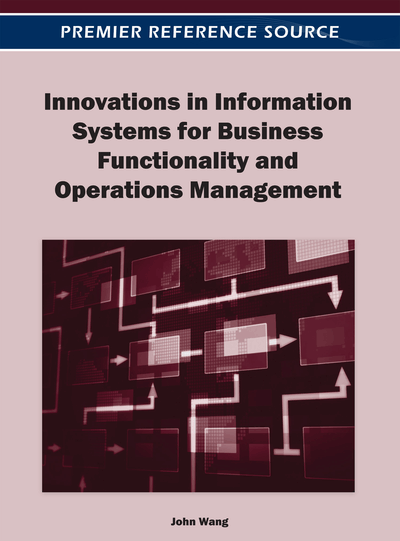# Solution of Correlated Multi-Response Optimization Problem: Case Study in Submerged Arc Welding

DOI: 10.4018/978-1-4666-0933-4.ch004

## Abstract

This paper highlights an integrated approach to solve the correlated multi-response optimization problem through a case study in submerged arc welding (SAW). The proposed approach has been presented to overcome different limitations and drawbacks of existing optimization techniques available in literature. Traditional Taguchi optimization technique is based under the assumption that quality responses are independent to each other; however, this assumption may not always be valid. A common trend in the solution of a multi-objective optimization problem is to convert these multi-objectives into an equivalent single objective function. While deriving this equivalent objective function, different weightage are assigned to different responses according to their relative priority. In this regard, it seems that no specific guideline is available for assigning individual response weighs. To avoid this, Principal Component Analysis (PCA) has been adopted to eliminate correlation among individual desirability values and to calculate uncorrelated quality indices that have been aggregated to calculate overall grey relational grade. This study combines PCA, Desirability Function (DF) approach, and grey relation theory to the entropy measurement technique. Finally, the Taguchi method has been used to derive optimal process environment capable of producing desired weld quality related to bead geometry.
Chapter Preview
Top

## Introduction

Literature depicts that, there are different approaches to tackle simulation modeling, prediction and multi-objective optimization problems in various fields in production engineering. The common approaches include Response Surface Methodology (RSM), (Murugan & Gunaraj, 2005), Artificial Neural Network (ANN), Genetic Algorithm (GA), Fuzzy regression, (Wang & Jean, 2006) and Desirability Function (DF), (Asiabanpour et al., 2004; Wu, 2005).

Gunaraj and Murugan, October 2000; November 2000 developed a model using the five level factorial technique to relate the important process control variables–welding voltage, wire feed rate, welding speed and nozzle to plate distance–to a few important bead quality parameters–penetration, reinforcement, bead width, total volume of the weld bead and dilution. They highlighted quantitatively as well as graphically the main effect and interactive effect of the process control variables on important bead geometry parameters.

Kim et al. (2005) developed an intelligent system of artificial neural network in GMA welding process using MATLAB/SIMULINK software. Based on multiple regressions and neural network, the mathematical models were derived from extensive experiments with different welding parameters and complex geometrical features. Their developed system was capable of receiving the desired weld dimensions as input and selecting the optimal welding parameters as outputs. Kim et al., 2003 applied an intelligent system for the determination of welding parameters for each pass and welding position, for pipeline welding, based on one database and a finite element method (FEM) model, and on two back-propagation (BP) neural network models and a corrective neural network model (CNN).

Xue et al. (2005) reported the possibilities of the fuzzy regression method in modeling the bead width in the robotic arc-welding process. In their paper, they developed a model for proper prediction of the process variables for obtaining the optimal bead width. Sathiya et al. (2004) proposed a method to decide near optimal settings of the process parameters using Genetic Algorithm to optimize weld quality in friction welding. Correia et al. (2004) stated about the possibility of using Genetic Algorithm as a method to decide near-optimal parameter setting of a GMAW process.

## Complete Chapter List

Search this Book:
Reset